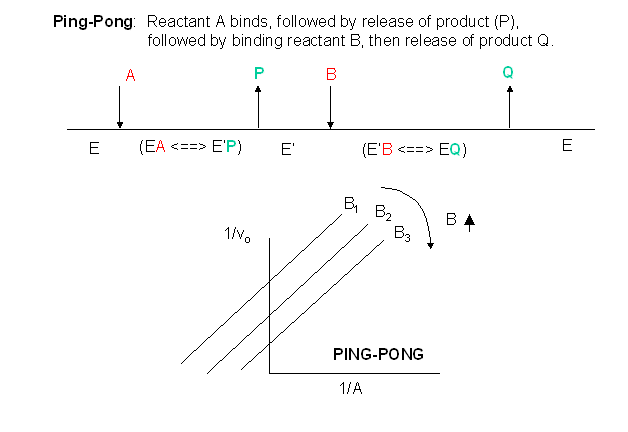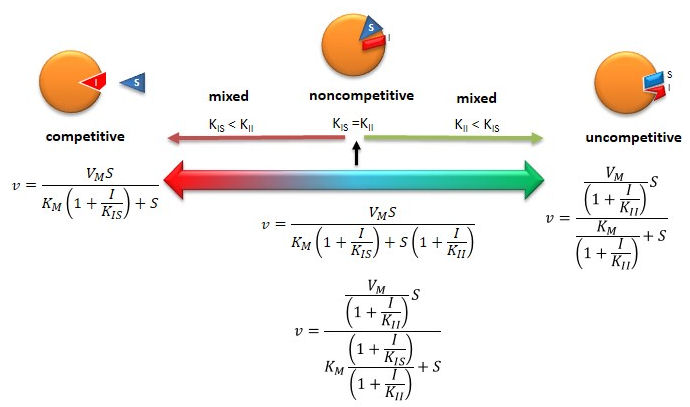# C4: Noncompetitive and Mixed Inhibition

$$\newcommand{\vecs}{\overset { \rightharpoonup} {\mathbf{#1}} }$$ $$\newcommand{\vecd}{\overset{-\!-\!\rightharpoonup}{\vphantom{a}\smash {#1}}}$$$$\newcommand{\id}{\mathrm{id}}$$ $$\newcommand{\Span}{\mathrm{span}}$$ $$\newcommand{\kernel}{\mathrm{null}\,}$$ $$\newcommand{\range}{\mathrm{range}\,}$$ $$\newcommand{\RealPart}{\mathrm{Re}}$$ $$\newcommand{\ImaginaryPart}{\mathrm{Im}}$$ $$\newcommand{\Argument}{\mathrm{Arg}}$$ $$\newcommand{\norm}{\| #1 \|}$$ $$\newcommand{\inner}{\langle #1, #2 \rangle}$$ $$\newcommand{\Span}{\mathrm{span}}$$ $$\newcommand{\id}{\mathrm{id}}$$ $$\newcommand{\Span}{\mathrm{span}}$$ $$\newcommand{\kernel}{\mathrm{null}\,}$$ $$\newcommand{\range}{\mathrm{range}\,}$$ $$\newcommand{\RealPart}{\mathrm{Re}}$$ $$\newcommand{\ImaginaryPart}{\mathrm{Im}}$$ $$\newcommand{\Argument}{\mathrm{Arg}}$$ $$\newcommand{\norm}{\| #1 \|}$$ $$\newcommand{\inner}{\langle #1, #2 \rangle}$$ $$\newcommand{\Span}{\mathrm{span}}$$

Reversible noncompetitive inhibition occurs when I binds to both E and ES. We will look at only the special case in which the dissociation constants of $$I$$ for $$E$$ and $$ES$$ are the same. This is called noncompetiive inhibition. It is quite rare as it would be difficult to imagine a large inhibitor which inhibits the turnover of bound substrate having no effect on binding of $$S$$ to $$E$$. However covalent interaction of protons with both E and ES can lead to noncompetitive inhibition. In the more general case, the $$K_d$$'s are different, and the inhibition is called mixed. Since inhibition occurs, we will hypothesize that ESI can not form product. It is a dead end complex which has only one fate, to return to $$ES$$ or $$EI$$. This is illustrated in the chemical equations and in the molecular cartoon below.Let us assume for ease of equation derivation that I binds reversibly to E with a dissociation constant of Kis (as we denoted for competitive inhibition) and to ES with a dissociation constant $$K_{ii}$$ (as we noted for uncompetitive inhibition). Assume for noncompetitive inhibition that Kis = Kii. A look at the top mechanism shows that in the presence of I, as S increases to infinity, not all of $$E$$ is converted to $$ES$$. That is, there is a finite amount of ESI, even at infinite S. Now remember that

$V_m = k_{cat}E_o$

if and only if all $$E$$ is in the form $$ES$$. Under these conditions, the apparent $$V_m$$, $$V_{m,app}$$ is less than the real $$V_m$$ without inhibitor. In contrast, the apparent Km, $$K_{m,app}$$, will not change since I binds to both $$E$$ and $$ES$$ with the same affinity, and hence will not perturb that equilibrium, as deduced from Le Chatelier's principle. The double reciprocal plot (Lineweaver Burk plot) offers a great way to visualize the inhibition. In the presence of I, just Vm will decrease. Therefore, -1/Km, the x-intercept will stay the same, and $$1/V_m$$ will get more positive. Therefore the plots will consists of a series of lines intersecting on the x axis, which is the hallmark of noncompetitive inhibition. You should be able to figure out how the plots would appear if $$K_{is}$$ is different from $$K_{ii}$$ (mixed inhibition).

An equation, shown in the diagram above can be derived which shows the effect of the noncompetitive inhibitor on the velocity of the reaction. In the denominator, Km is multiplied by $$1+I/K_{is}$$, and $$S$$ by $$1+I/K_{ii}$$. We would like to rearrange this equation to show how Km and Vm are affected by the inhibitor, not S, which obviously isn't. Rearranging the equation as shown above shows that

$K_{m,app} = \dfrac{K_m(1+I/K_{is})}{1+I/K_{ii}} = K_m$

when

$K_{is}=K_{ii}$

and

$V_{m,app} = \frac{V_m}{1+I/Kii}.$

This shows that the $$K_m$$ is unchanged and $$V_m$$ decreases as we predicted. The plot shows a series of lines intersecting on the x axis. Both the slope and the y intercept are changed, which are reflected in the names of the two dissociation constants, Kis and Kii. Note that if $$I$$ is zero, $$K_{m,app} = K_m$$ and $$V_{m,app} = V_m$$. Sometimes the $$K_{is}$$ and $$K_{ii}$$ inhibition dissociations constants are referred to as $$K_c$$ and $$K_u$$ (competitive and uncompetitive inhibition dissociation constants.Mixed (and non-)competitive inhibition (as shown by mechanism above) differ from competitive and uncompetiive inhibition in that the inhibitor binding is not simply a dead end reaction in which the inhibitor can only dissociate in a single reverse step. In the above equilibrium, $$S$$ can dissociate from $$ESI$$ to form $$EI$$ so the system may not be at equilibrium. With dead end steps, no flux of reactants occurs through the dead end complex so the equilibrium for the dead end step is not perturbed.

Other mechanisms can commonly give mixed inhibition. For example, the product released in a ping pong mechanism (discussed in the next chapter) can give mixed inhibition.If $$P$$, acting as a product inhibitor, can bind to two different forms of the enzyme ($$E'$$ and also $$E$$), it will act as an mixed inhibitor.Java Applet: Noncompetitive Inhibition4/26/13Wolfram Mathematica CDF Player - Mixed Inhibition v vs S curves; Kis and Kii called Kc and Ku (start sliders at high values) (free plugin required)4/26/13Wolfram Mathematica CDF Player - Mixed Inhibition v vs S curves (start sliders at high values) (free plugin required). Note where the inhibited and inhibited curves intersect at different values of Kis and Kii (in the graph termed Kc and Ku).

If you can apply Le Chatelier's principle, you should be able to draw the Lineweaver-Burk plots for any scenario of inhibition or even the opposite case, enzyme activation!Figure: Summary of Reversible Enzyme Inhibition